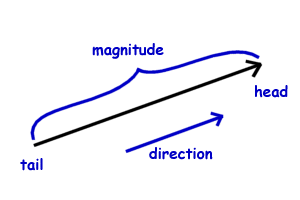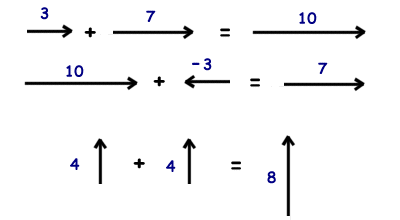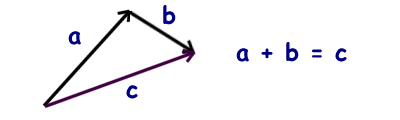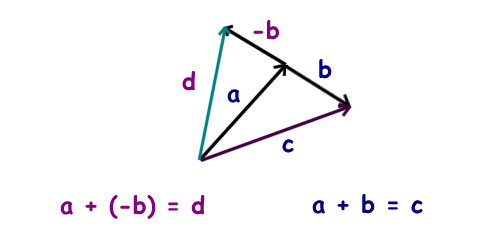Science >> Physics for Kids

# Physics for Kids

## Basic Vector Math

Vector Basics

A vector is a property that has both a magnitude and a direction. Vectors are drawn as an arrow with a tail and head. The length of the vector represents its magnitude.Vectors are written using a letter and boldface type. For example, you would have the vector a or the vector b. If you were just talking about the magnitude of the vector you would write the letter inside parallel lines like this: ||a||

Vectors can be added together to find out the resultant of both vectors (a + b = c). Both the directions and the magnitudes are combined when adding vectors. Here are some simple examples adding vectors that are in same direction or 180 degrees of the same direction (negative).What do we do when we are adding vectors that are not in the same direction?

One way to add vectors is using the head-to-tail method. In this method we put the tail of the additional vector at the end of the head of the previous vector. The resultant vector is the vector drawn from the tail of the first vector to the head of the last vector. See the example using two vectors below.Pythagorean Theorem

If the two vectors a and b form a 90 degree angle, we can use the Pythagorean Theorem to find the magnitude of the resultant vector c. You can go here to learn more about the Pythagorean Theorem.

In this case, the magnitude of the sum of the vectors a + b = c is a2 + b2 = c2.

Example problem:

Jim walks four miles north and then walks three miles east. What was the resultant distance if he had walked a straight line from the starting point to the end point?

Since Jim walked in two vectors, one to the north and one to the east, we can add these vectors together to get the answer. As north and east are at 90 degrees to each other we can use the Pythagorean Theorem.

c2 = a2 + b2
c2 = 32 + 42
c2 = 9 + 16
c2 = 25
c = 5

Commutative Law

The commutative law for vector addition states that it doesn't matter in which order the vectors are added together.

a + b = b + c

Associative Law

The associative law for vector addition states that when three or more vectors are added together, it doesn't matter which vectors are added together first.

(a + b) + d = a + (b + d)

Subtracting Vectors

When subtracting two vectors a - b, it is the same as adding the vectors a + (-b). The negative vector is the same magnitude, but is drawn in the opposite direction of the positive vector.More Physics Subjects on Motion, Work, and Energy

Science >> Physics for Kids